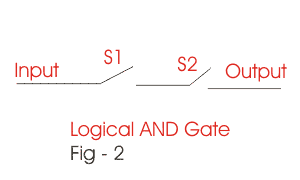# AND Operation | Logical AND Operation

This operation is also a very important in logical operation in digital electronics. In other words Logical AND operation is also known as Boolean AND operation. AND operation is different from OR operation. Like Logical OR operation this is also very useful in digital circuits and arithmetical solves. There are several I.Cs where the concept of Logical AND operation is used. Again to understand these types of operation we have to go through the truth table. In Boolean AND operation the sign used is (.). Here also we have two inputs and a single output. Now let us follow the truth table of AND operation.Here the outputs are quite different from OR operation. Traverse the table row by row. Firstly the inputs are A = 0 and B = 0 for which after logical AND operation the output is Y = 0. Again traverse to the next row, here the inputs are A = 0 and B = 1. The respective output is 0. Now the inputs are A = 1 and B = 0 and the output after AND operation is also 0.

 INPUT OUTPUT A B Y = A.B 0 0 0 0 1 0 1 0 0 1 1 1

Lastly when both A&B = 1 the output is 1. Thus we can find the difference of it from Boolean OR operation. Here 1 is received as output only when both the inputs are 1. When either of the input is 0 the output for Boolean AND operation is 0. Again this phenomenon can be under stand by simple switch circuit. To understand this Boolean operation let concentrate on fig. 2.
In the above figure two switches are connected in series i.e one by one. Now see if both the switches are opened no signal will be received in the output. i.e if S1 = 0 and S2 = 0 the output is also 0. Now make either of the input 1. When S1 = 1, S2 = 0 and when S2 = 1, S1 = 0. In both the cases one switch is closed but other is opened, thus no output signal is received. Output signal is received only when both the switches are closed i.e when s1 and s2 both are 1. Thus you can understand how Logical AND operation or Boolean AND operation works.

## Complement Logic

 INPUT OUTPUT A A’ 0 1 1 0

In Complement Logic on logic is enough. Here if the input is 1 the output will be 0 and if the input is 0 the output is 1. Thus you can say that it is totally different from AND operation and OR operation. The Complement Logic can be better understandable to you from the truth table given beside. Here the sign is (‘).

In logical complimentation as you can see that for the input 1 the respective output is 0 and vice versa. This operation phenomenon is also available in I.Cs which has a wide range of implementation in our real life.

Want To Learn Faster? 🎓
Get electrical articles delivered to your inbox every week.
No credit card required—it’s 100% free.